## Set Theory

It is natural for us to classify items into groups, or sets, and consider how those sets overlap with each other. We can use these sets understand relationships between groups, and to analyze survey data.

## Basics

An art collector might own a collection of paintings, while a music lover might keep a collection of CDs. Any collection of items can form a set.

### Set

A set is a collection of distinct objects, called elements of the set

A set can be defined by describing the contents, or by listing the elements of the set, enclosed in curly brackets.

### Example 1

Some examples of sets defined by describing the contents:

1. The set of all even numbers
2. The set of all books written about travel to Chile

Some examples of sets defined by listing the elements of the set:

1. {1, 3, 9, 12}
2. {red, orange, yellow, green, blue, indigo, purple}

A set simply specifies the contents; order is not important. The set represented by {1, 2, 3} is equivalent to the set {3, 1, 2}.

### Notation

Commonly, we will use a variable to represent a set, to make it easier to refer to that set later.

The symbol ∈ means “is an element of”.

A set that contains no elements, { }, is called the empty set and is notated ∅

### Example 2

Let A = {1, 2, 3, 4}

To notate that 2 is element of the set, we’d write 2 ∈ A

Sometimes a collection might not contain all the elements of a set. For example, Chris owns three Madonna albums. While Chris’s collection is a set, we can also say it is a subset of the larger set of all Madonna albums.

### Subset

A subset of a set A is another set that contains only elements from the set A, but may not contain all the elements of A.

If B is a subset of A, we write BA

A proper subset is a subset that is not identical to the original set—it contains fewer elements.

If B is a proper subset of A, we write BA

### Example 3

Consider these three sets:

A = the set of all even numbers
B = {2, 4, 6}
C = {2, 3, 4, 6}

Here BA since every element of B is also an even number, so is an element of A.

More formally, we could say BA since if x ∈ B, then x A.

It is also true that BC.

C is not a subset of A, since C contains an element, 3, that is not contained in A

### Example 4

Suppose a set contains the plays “Much Ado About Nothing,” “MacBeth,” and “A Midsummer’s Night Dream.” What is a larger set this might be a subset of?

There are many possible answers here. One would be the set of plays by Shakespeare. This is also a subset of the set of all plays ever written. It is also a subset of all British literature.

### Try It Now

The set A = {1, 3, 5}. What is a larger set this might be a subset of?

## Union, Intersection, and Complement

Commonly sets interact. For example, you and a new roommate decide to have a house party, and you both invite your circle of friends. At this party, two sets are being combined, though it might turn out that there are some friends that were in both sets.

### Union, Intersection, and Complement

The union of two sets contains all the elements contained in either set (or both sets). The union is notated A B. More formally, x A B if x A or x B (or both)

The intersection of two sets contains only the elements that are in both sets. The intersection is notated A B. More formally, x A B if x A and x B.

The complement of a set A contains everything that is not in the set A. The complement is notated A’, or Ac, or sometimes ~A.

### Example 5

Consider the sets:

A = {red, green, blue}
B = {red, yellow, orange}
C = {red, orange, yellow, green, blue, purple}

Find the following:

1. Find A B
2. Find A B
3. Find Ac C

1. The union contains all the elements in either set: A B = {red, green, blue, yellow, orange} Notice we only list red once.
2. The intersection contains all the elements in both sets: A B = {red}
3. Here we’re looking for all the elements that are not in set A and are also in CAc C = {orange, yellow, purple}

### Try It Now

Using the sets from the previous example, find A C and Bc  A

Notice that in the example above, it would be hard to just ask for Ac, since everything from the color fuchsia to puppies and peanut butter are included in the complement of the set. For this reason, complements are usually only used with intersections, or when we have a universal set in place.

### Universal Set

A universal set is a set that contains all the elements we are interested in. This would have to be defined by the context.

A complement is relative to the universal set, so Ac contains all the elements in the universal set that are not in A.

### Example 6

1. If we were discussing searching for books, the universal set might be all the books in the library.
3. If you were working with sets of numbers, the universal set might be all whole numbers, all integers, or all real numbers

### Example 7

Suppose the universal set is U = all whole numbers from 1 to 9. If A = {1, 2, 4}, then Ac = {3, 5, 6, 7, 8, 9}.

As we saw earlier with the expression Ac  C, set operations can be grouped together. Grouping symbols can be used like they are with arithmetic – to force an order of operations.

### Example 8

Suppose H = {cat, dog, rabbit, mouse}, F = {dog, cow, duck, pig, rabbit}, and W = {duck, rabbit, deer, frog, mouse}

1. Find (H F) ⋃ W
2. Find H ⋂ (F W)
3. Find (H F)c W

#### Solutions

1. We start with the intersection: H F = {dog, rabbit}. Now we union that result with W: (H F) ⋃ W = {dog, duck, rabbit, deer, frog, mouse}
2. We start with the union: F W = {dog, cow, rabbit, duck, pig, deer, frog, mouse}. Now we intersect that result with H: H ⋂ (F W) = {dog, rabbit, mouse}
3. We start with the intersection: H F = {dog, rabbit}. Now we want to find the elements of W that are not in H F. (H F)c ⋂ W = {duck, deer, frog, mouse}

## Venn Diagrams

To visualize the interaction of sets, John Venn in 1880 thought to use overlapping circles, building on a similar idea used by Leonhard Euler in the eighteenth century. These illustrations now called Venn Diagrams.

### Venn Diagram

A Venn diagram represents each set by a circle, usually drawn inside of a containing box representing the universal set. Overlapping areas indicate elements common to both sets.

Basic Venn diagrams can illustrate the interaction of two or three sets.

### Example 9

Create Venn diagrams to illustrate A B, A B, and Ac B

A B contains all elements in either set.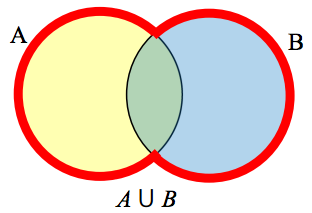A B contains only those elements in both sets—in the overlap of the circles.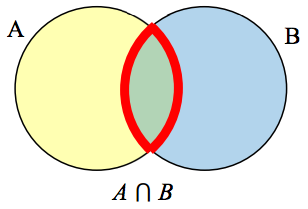Ac will contain all elements not in the set A. Ac  B will contain the elements in set B that are not in set A.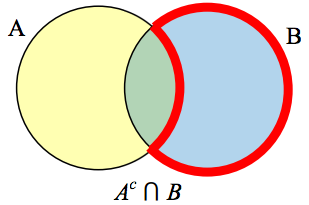### Example 10

Use a Venn diagram to illustrate (H F)c ⋂ W

We’ll start by identifying everything in the set H F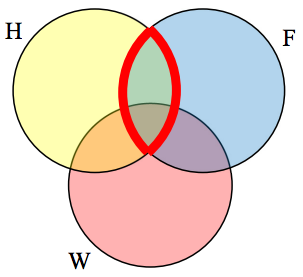Now, (H F)c ⋂ W will contain everything not in the set identified above that is also in set W.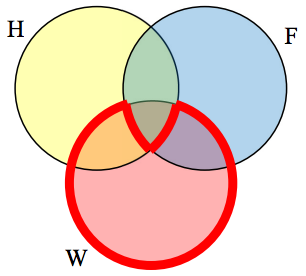### Example 11

Create an expression to represent the outlined part of the Venn diagram shown.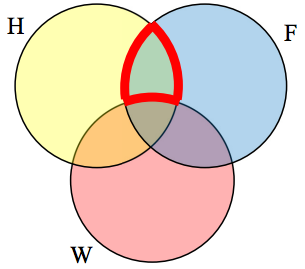The elements in the outlined set are in sets H and F, but are not in set W. So we could represent this set as H F Wc

### Try It Now

Create an expression to represent the outlined portion of the Venn diagram shown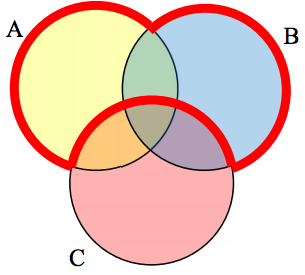## Cardinality

Often times we are interested in the number of items in a set or subset. This is called the cardinality of the set.

### Cardinality

The number of elements in a set is the cardinality of that set.

The cardinality of the set A is often notated as |A| or n(A)

### Example 12

Let A = {1, 2, 3, 4, 5, 6} and B = {2, 4, 6, 8}.

What is the cardinality of B? A B, A B?

The cardinality of B is 4, since there are 4 elements in the set.

The cardinality of A B is 7, since A B = {1, 2, 3, 4, 5, 6, 8}, which contains 7 elements.

The cardinality of A B is 3, since A B = {2, 4, 6}, which contains 3 elements.

### Example 13

What is the cardinality of P = the set of English names for the months of the year?

The cardinality of this set is 12, since there are 12 months in the year.

Sometimes we may be interested in the cardinality of the union or intersection of sets, but not know the actual elements of each set. This is common in surveying.

### Example 14

A survey asks 200 people “What beverage do you drink in the morning”, and offers choices:

• Tea only
• Coffee only
• Both coffee and tea

Suppose 20 report tea only, 80 report coffee only, 40 report both.   How many people drink tea in the morning? How many people drink neither tea or coffee?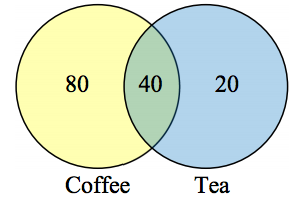This question can most easily be answered by creating a Venn diagram. We can see that we can find the people who drink tea by adding those who drink only tea to those who drink both: 60 people.

We can also see that those who drink neither are those not contained in the any of the three other groupings, so we can count those by subtracting from the cardinality of the universal set, 200.

200 – 20 – 80 – 40 = 60 people who drink neither.

### Example 15

A survey asks: “Which online services have you used in the last month?”

• Have used both

The results show 40% of those surveyed have used Twitter, 70% have used Facebook, and 20% have used both. How many people have used neither Twitter or Facebook?

Let T be the set of all people who have used Twitter, and F be the set of all people who have used Facebook. Notice that while the cardinality of F is 70% and the cardinality of T is 40%, the cardinality of FT is not simply 70% + 40%, since that would count those who use both services twice. To find the cardinality of FT, we can add the cardinality of F and the cardinality of T, then subtract those in intersection that we’ve counted twice. In symbols,

n(FT) = n(F) + n(T) – n(FT)
n(FT) = 70% + 40% – 20% = 90%

Now, to find how many people have not used either service, we’re looking for the cardinality of (FT)c . Since the universal set contains 100% of people and the cardinality of FT = 90%, the cardinality of (FT)c must be the other 10%.

The previous example illustrated two important properties

### Cardinality properties

n(AB) = n(A) + n(B) – n(AB)

n(Ac) = n(U) – n(A)

Notice that the first property can also be written in an equivalent form by solving for the cardinality of the intersection:

n(AB) = n(A) + n(B) – n(AB)

### Example 16

Fifty students were surveyed, and asked if they were taking a social science (SS), humanities (HM) or a natural science (NS) course the next quarter.

 21 were taking a SS course 26 were taking a HM course 19 were taking a NS course 9 were taking SS and HM 7 were taking SS and NS 10 were taking HM and NS 3 were taking all three 7 were taking none

How many students are only taking a SS course?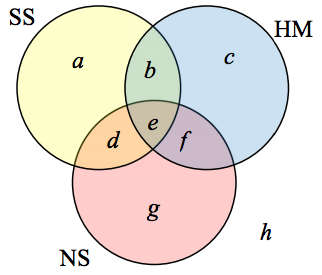It might help to look at a Venn diagram. From the given data, we know that there are 3 students in region e and 7 students in region h.

Since 7 students were taking a SS and NS course, we know that n(d) + n(e) = 7. Since we know there are 3 students in region 3, there must be 7 – 3 = 4 students in region d.

Similarly, since there are 10 students taking HM and NS, which includes regions e and f, there must be 10 – 3 = 7 students in region f.

Since 9 students were taking SS and HM, there must be 9 – 3 = 6 students in region b.

Now, we know that 21 students were taking a SS course. This includes students from regions a, b, d, and e. Since we know the number of students in all but region a, we can determine that 21 – 6 – 4 – 3 = 8 students are in region a.

8 students are taking only a SS course.

### Try It Now

One hundred fifty people were surveyed and asked if they believed in UFOs, ghosts, and Bigfoot.

 43 believed in UFOs 44 believed in ghosts 25 believed in Bigfoot 10 believed in UFOs and ghosts 8 believed in ghosts and Bigfoot 5 believed in UFOs and Bigfoot 2 believed in all three

How many people surveyed believed in at least one of these things?